# 角色动画研究 —— 使用雅可比矩阵(Jacobian)来结算IK

## 雅可比矩阵

y1=f1(x1,x2,x3,x4,x5,x6)

y2=f2(x1,x2,x3,x4,x5,x6)

y3=f3(x1,x2,x3,x4,x5,x6)

y4=f4(x1,x2,x3,x4,x5,x6)

y5=f5(x1,x2,x3,x4,x5,x6)

y6=f6(x1,x2,x3,x4,x5,x6)

dyi=fix1dx1+fix2dx2+fix3dx3+fix4dx4+fix5dx5+fix6dx6

dY=FXdX

Y˙=J(X)X˙

## 雅可比矩阵与动力学

### 雅可比矩阵的解释

Y=[px py pz αx αy αz]T

V=Y˙=J(θ)θ˙

V=[vx vy vz ωx ωy ωz]

θ˙ $\dot{\theta}$指代的是各个关节的速度，在上面的方程中，是未知的。

θ˙=[θ1˙ θ2˙ .... θn˙ ]T

J $J$就是雅可比矩阵，指代的就是在当前的pose下，各个关节的变化导致的终端骨节的变化的矩阵。

J=pxθ1pyθ1pzθ1...αzθ1pxθ2pyθ2pzθ2...αzθ2...............pxθnpyθnpzθn...αzθn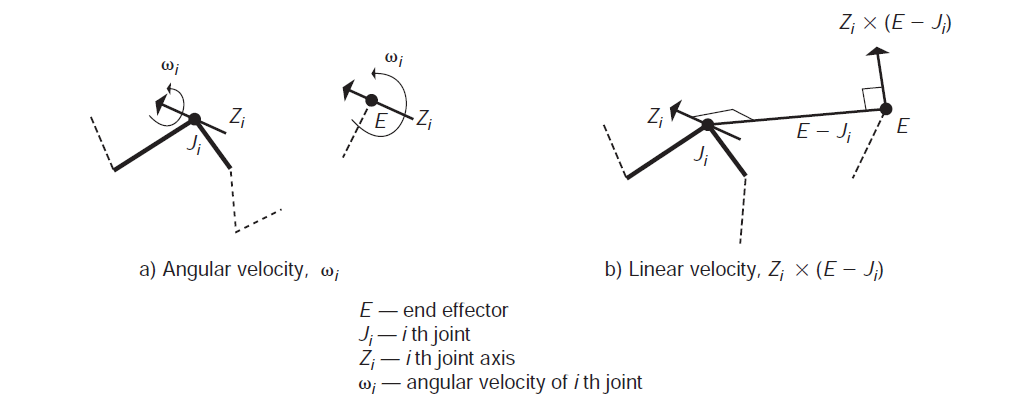### 雅可比矩阵示例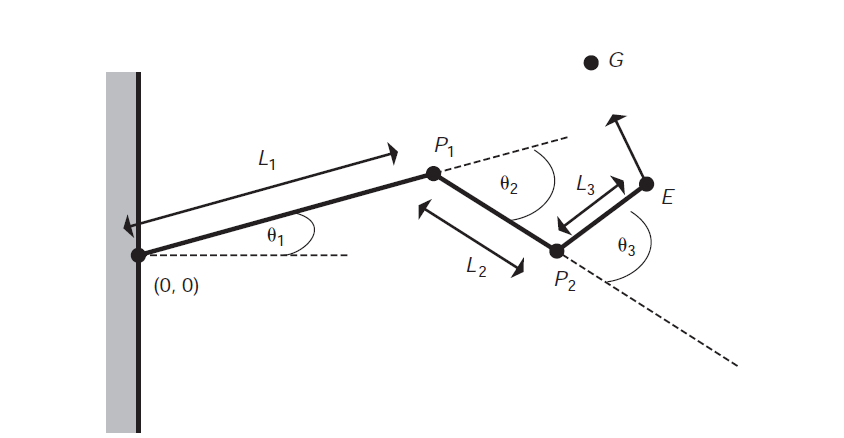V=(GE)x(GE)y(GE)z

J=((0,0,1)×E)x   ((0,0,1)×(EP1))x   ((0,0,1)×(EP2))x((0,0,1)×E)y   ((0,0,1)×(EP1))y   ((0,0,1)×(EP2))y((0,0,1)×E)z   ((0,0,1)×(EP1))z   ((0,0,1)×(EP2))z

## 矩阵的求解

V=Jθ˙

J1V=θ˙

### 广义逆

A+A=In       (nm)

AA+=Im       (mn)

A+ $A^+$的值为：

A+=(ATA)1AT       (nm)

A+=AT(AAT)1       (mn)

### 推导过程

V=Jθ˙

J+V=J+Jθ˙

J+V=JT(JJT)1Jθ˙

(JJT)1 $(JJ^T)^{-1}$展开为 (JT)1J1 $(J^T)^{-1}J^{-1}$，会发现右边的公式直接被消掉，也就是说：
JT(JJT)1V=θ˙

(JJT)β=V

JTβ=θ˙

## 骨节迭代Integration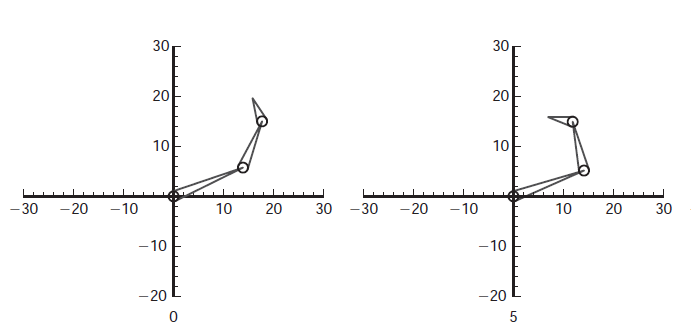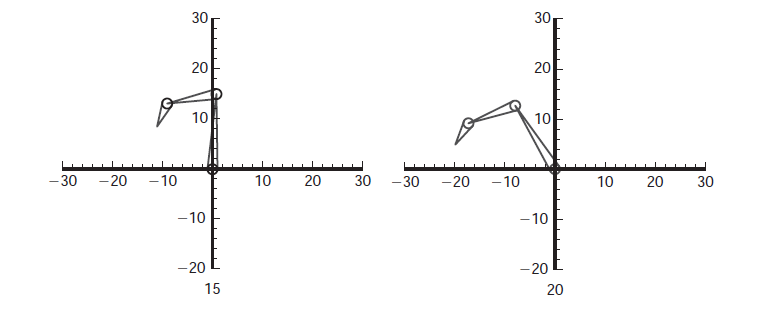θ˙=JT(JJT+λ2I)1V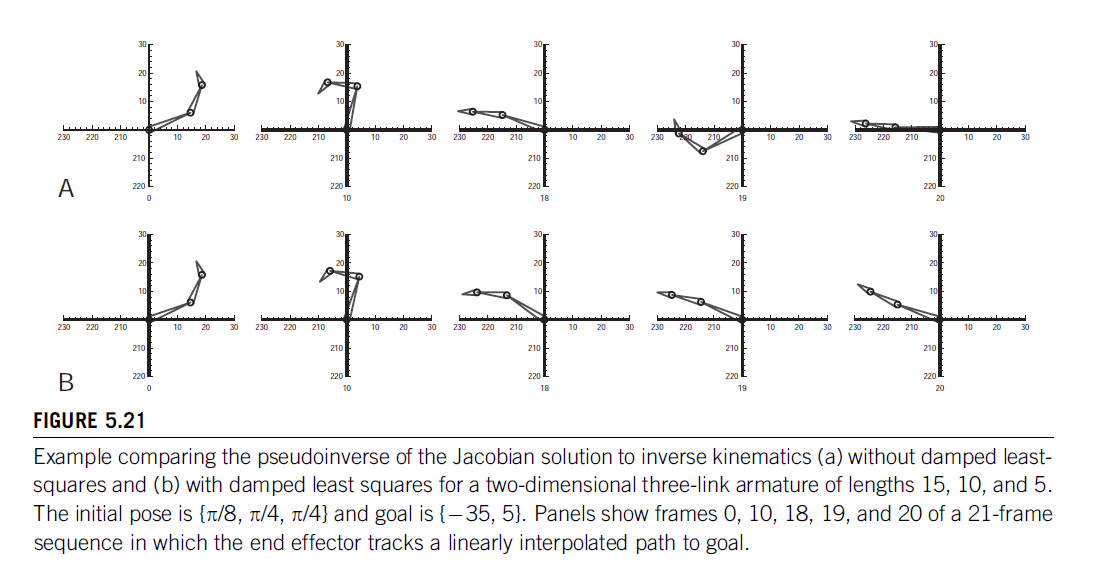## 使用雅可比矩阵的转置而不是逆

θ˙=αJTV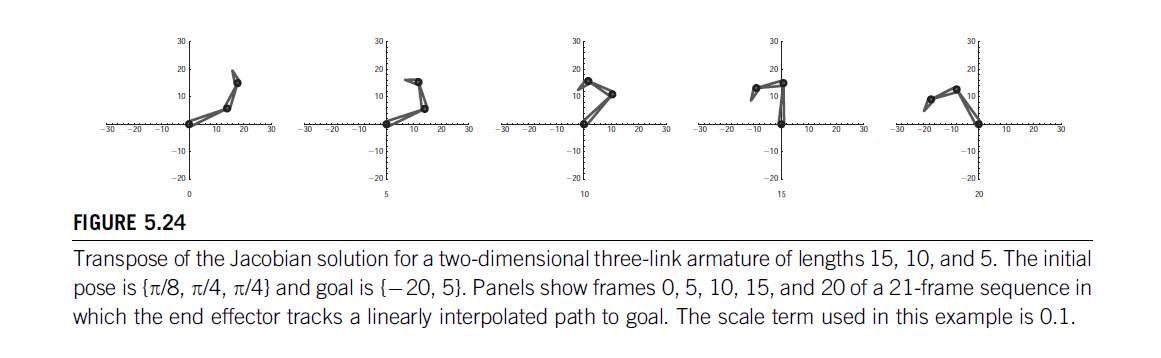## 额外收获

<全文完>11-02
04-30
02-18120
02-19291
09-041940
10-133万+
08-131301
©️2020 CSDN 皮肤主题: 大白 设计师:CSDN官方博客